Скачать презентацию Problem Solving in Chemical Engineering with Numerical Methods

52df6c7921a8a4a216d05a62faf673f4.ppt

• Количество слайдов: 22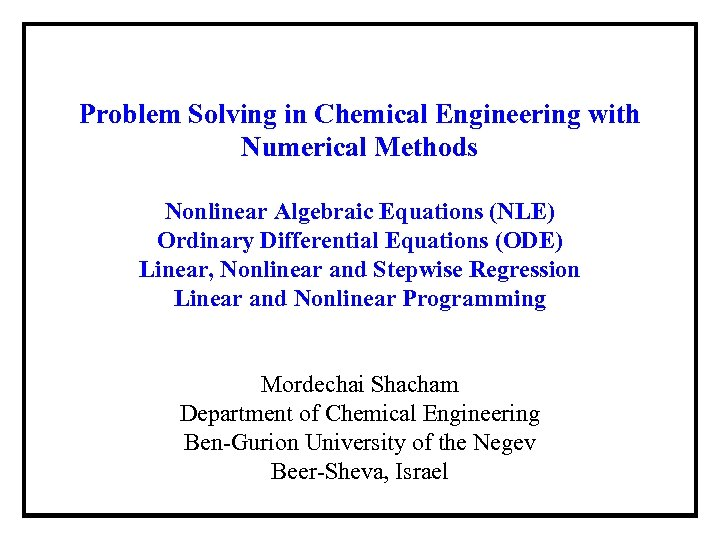Problem Solving in Chemical Engineering with Numerical Methods Nonlinear Algebraic Equations (NLE) Ordinary Differential Equations (ODE) Linear, Nonlinear and Stepwise Regression Linear and Nonlinear Programming Mordechai Shacham Department of Chemical Engineering Ben-Gurion University of the Negev Beer-Sheva, Israel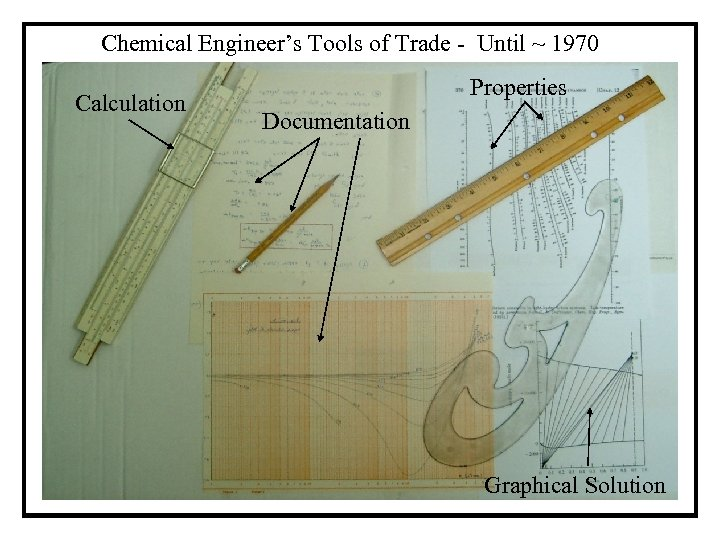Chemical Engineer’s Tools of Trade - Until ~ 1970 Calculation Properties Documentation Graphical Solution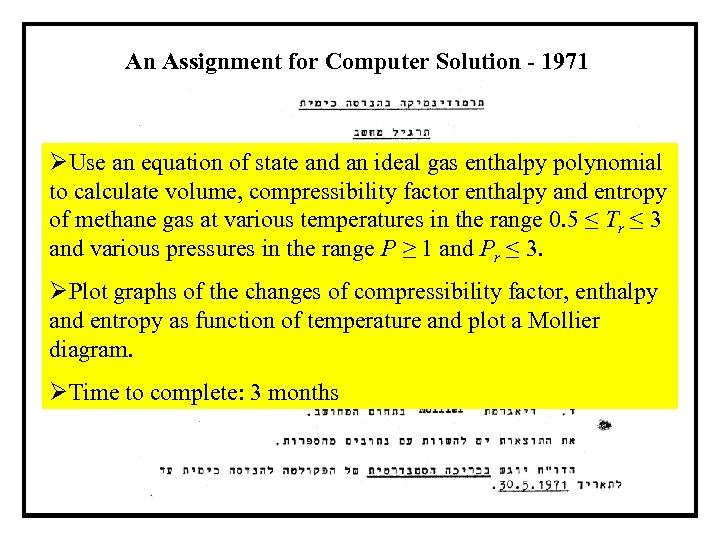An Assignment for Computer Solution - 1971 ØUse an equation of state and an ideal gas enthalpy polynomial to calculate volume, compressibility factor enthalpy and entropy of methane gas at various temperatures in the range 0. 5 ≤ Tr ≤ 3 and various pressures in the range P ≥ 1 and Pr ≤ 3. ØPlot graphs of the changes of compressibility factor, enthalpy and entropy as function of temperature and plot a Mollier diagram. ØTime to complete: 3 months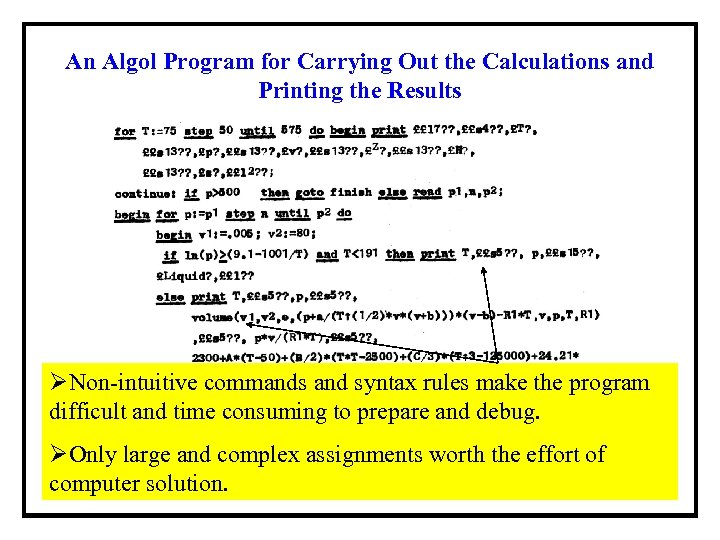An Algol Program for Carrying Out the Calculations and Printing the Results ØNon-intuitive commands and syntax rules make the program difficult and time consuming to prepare and debug. ØOnly large and complex assignments worth the effort of computer solution.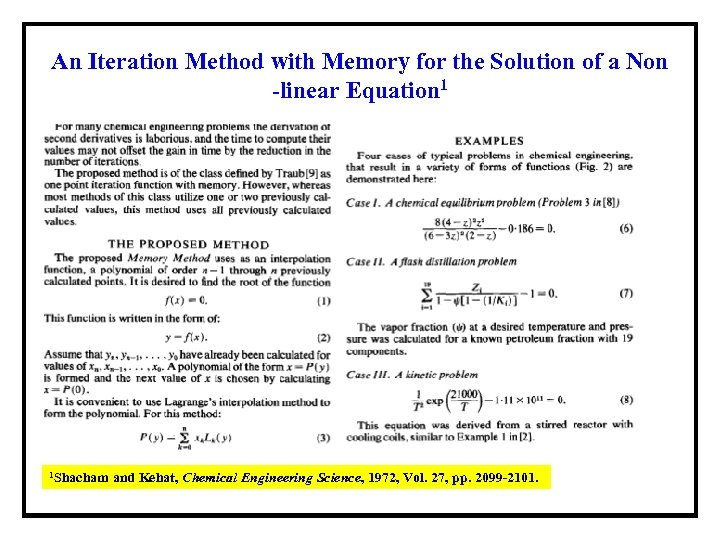An Iteration Method with Memory for the Solution of a Non -linear Equation 1 1 Shacham and Kehat, Chemical Engineering Science, 1972, Vol. 27, pp. 2099 -2101.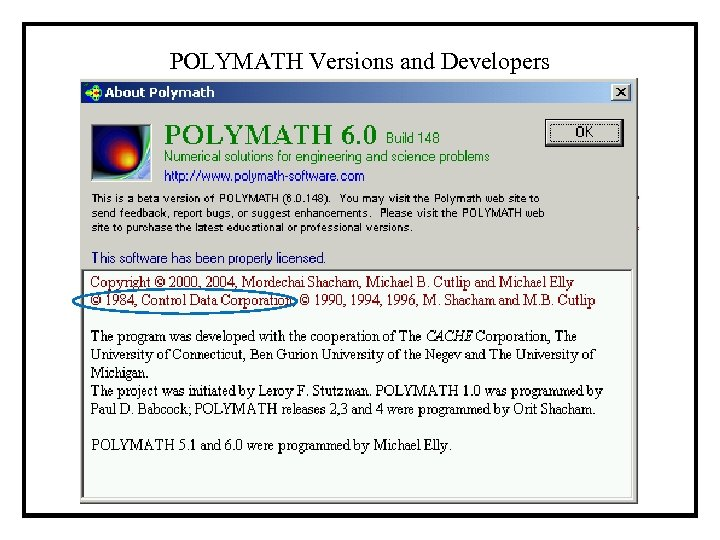POLYMATH Versions and Developers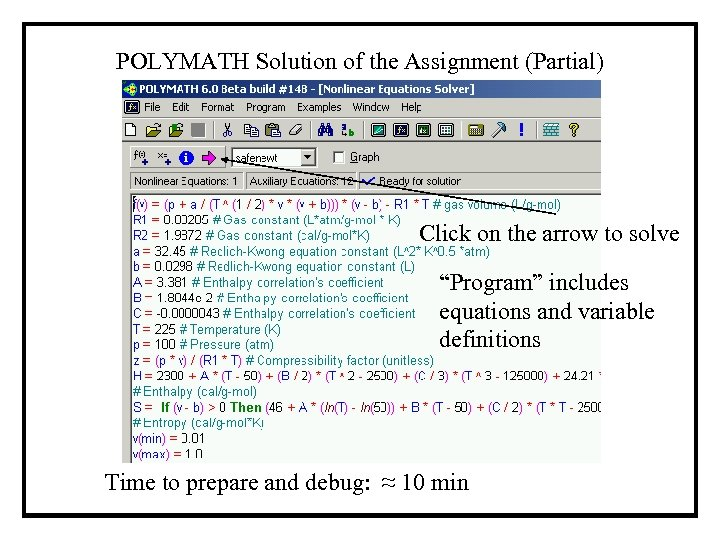POLYMATH Solution of the Assignment (Partial) Click on the arrow to solve “Program” includes equations and variable definitions Time to prepare and debug: ≈ 10 min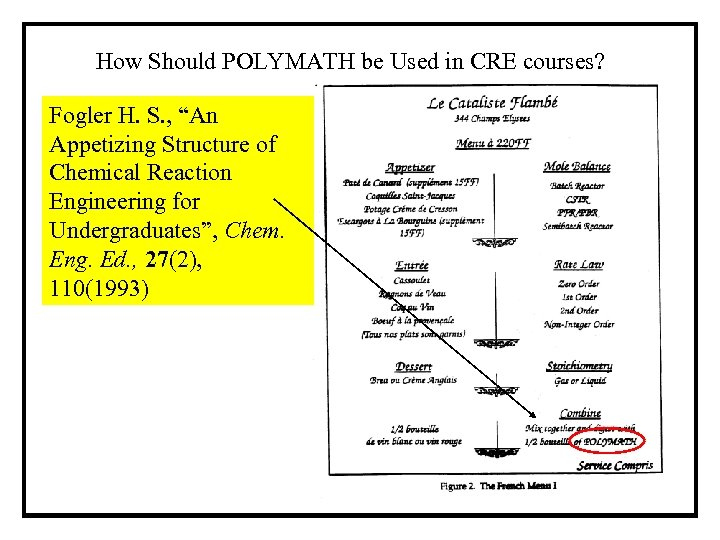How Should POLYMATH be Used in CRE courses? Fogler H. S. , “An Appetizing Structure of Chemical Reaction Engineering for Undergraduates”, Chem. Eng. Ed. , 27(2), 110(1993)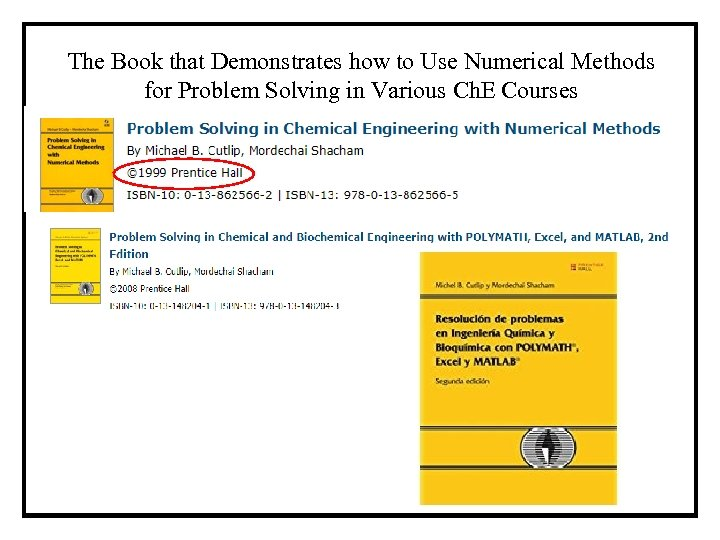The Book that Demonstrates how to Use Numerical Methods for Problem Solving in Various Ch. E Courses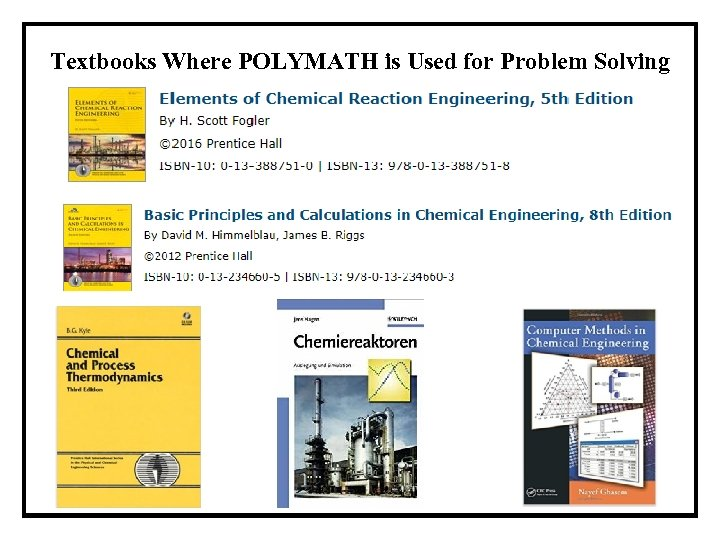Textbooks Where POLYMATH is Used for Problem Solving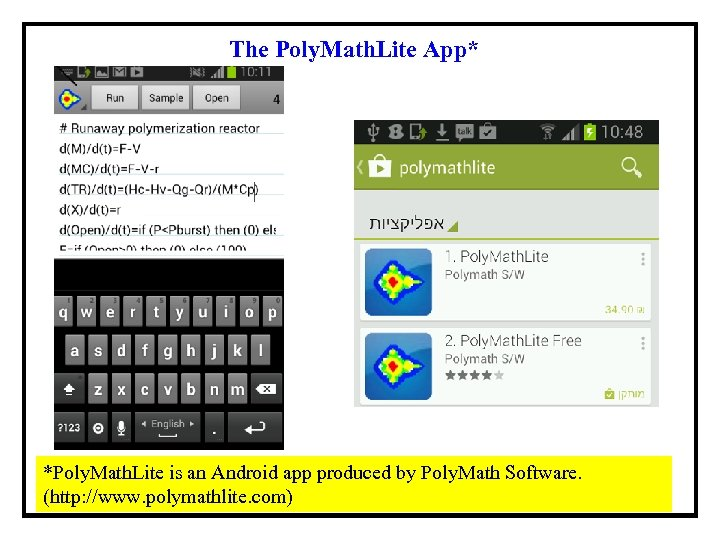The Poly. Math. Lite App* *Poly. Math. Lite is an Android app produced by Poly. Math Software. (http: //www. polymathlite. com)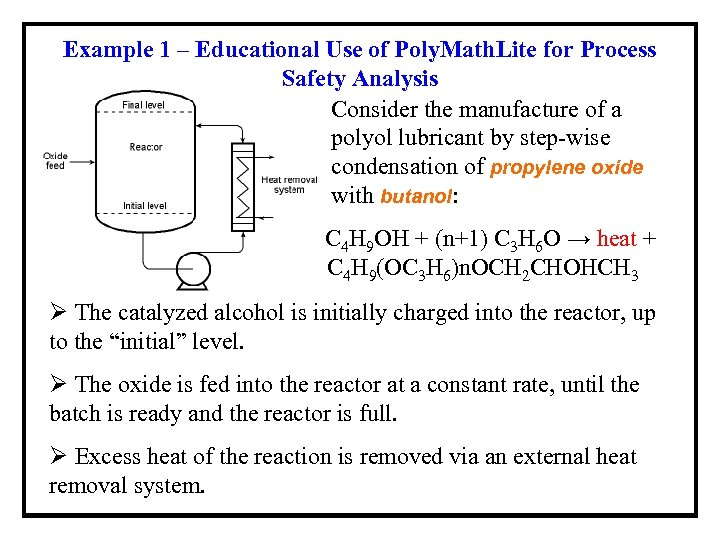Example 1 – Educational Use of Poly. Math. Lite for Process Safety Analysis Consider the manufacture of a polyol lubricant by step-wise condensation of propylene oxide with butanol: C 4 H 9 OH + (n+1) C 3 H 6 O → heat + C 4 H 9(OC 3 H 6)n. OCH 2 CHOHCH 3 Ø The catalyzed alcohol is initially charged into the reactor, up to the “initial” level. Ø The oxide is fed into the reactor at a constant rate, until the batch is ready and the reactor is full. Ø Excess heat of the reaction is removed via an external heat removal system.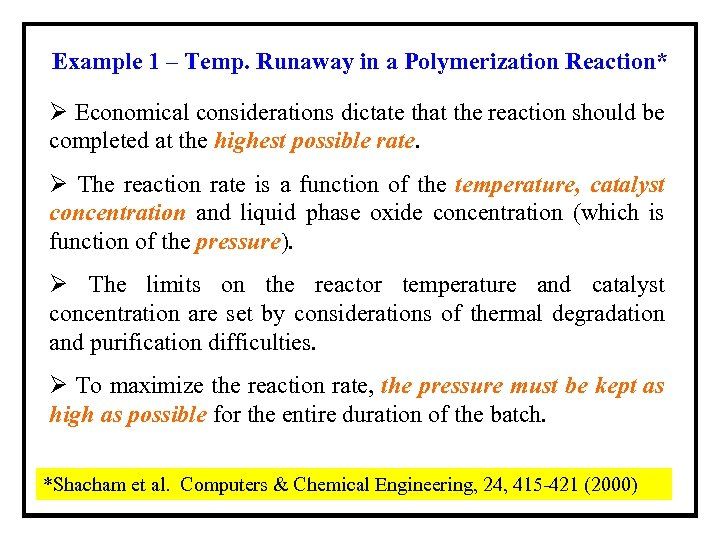Example 1 – Temp. Runaway in a Polymerization Reaction* Ø Economical considerations dictate that the reaction should be completed at the highest possible rate. Ø The reaction rate is a function of the temperature, catalyst concentration and liquid phase oxide concentration (which is function of the pressure). Ø The limits on the reactor temperature and catalyst concentration are set by considerations of thermal degradation and purification difficulties. Ø To maximize the reaction rate, the pressure must be kept as high as possible for the entire duration of the batch. *Shacham et al. Computers & Chemical Engineering, 24, 415 -421 (2000)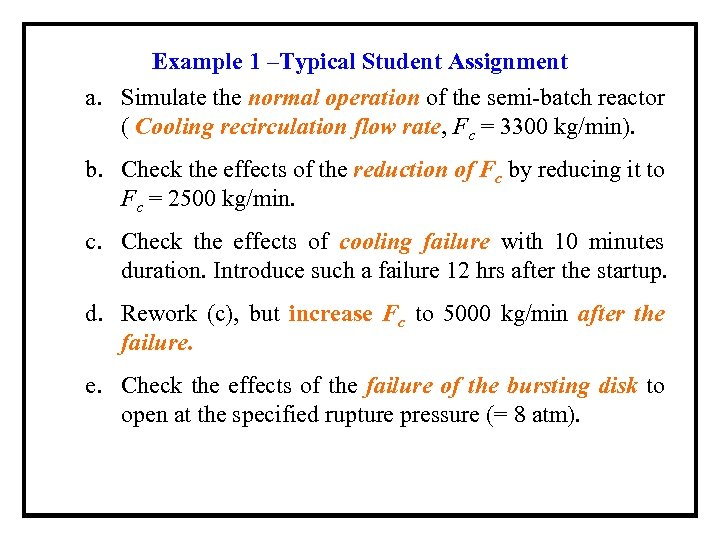Example 1 –Typical Student Assignment a. Simulate the normal operation of the semi-batch reactor ( Cooling recirculation flow rate, Fc = 3300 kg/min). b. Check the effects of the reduction of Fc by reducing it to Fc = 2500 kg/min. c. Check the effects of cooling failure with 10 minutes duration. Introduce such a failure 12 hrs after the startup. d. Rework (c), but increase Fc to 5000 kg/min after the failure. e. Check the effects of the failure of the bursting disk to open at the specified rupture pressure (= 8 atm).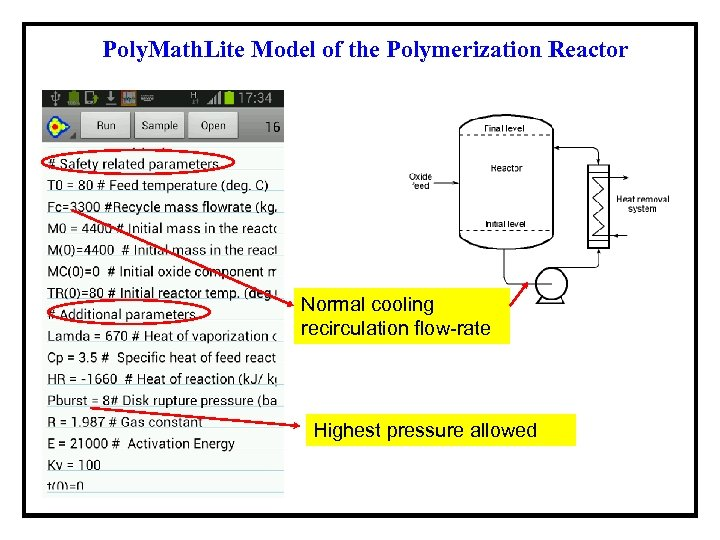Poly. Math. Lite Model of the Polymerization Reactor Normal cooling recirculation flow-rate Highest pressure allowed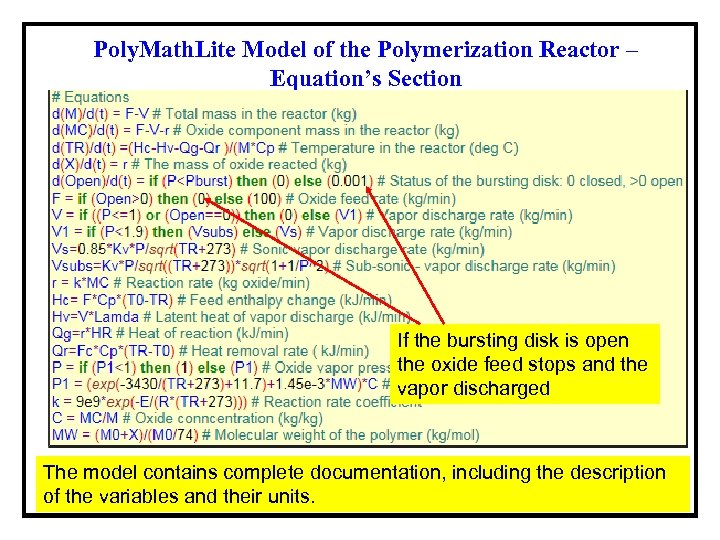Poly. Math. Lite Model of the Polymerization Reactor – Equation’s Section If the bursting disk is open the oxide feed stops and the vapor discharged The model contains complete documentation, including the description of the variables and their units.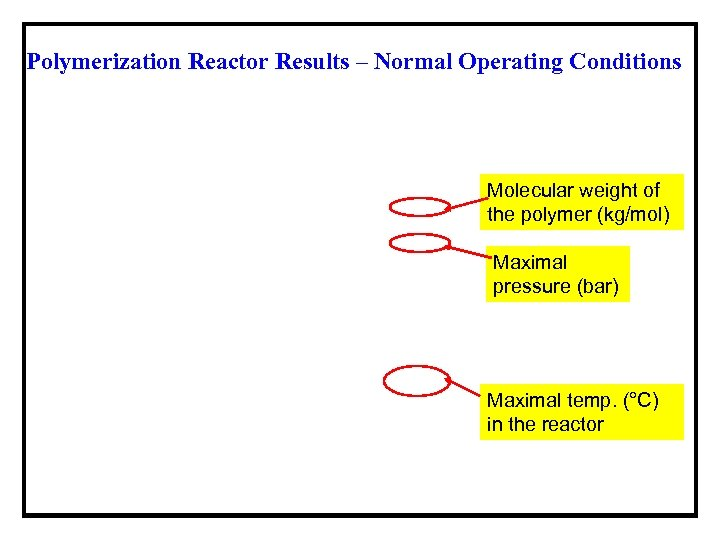Polymerization Reactor Results – Normal Operating Conditions Molecular weight of the polymer (kg/mol) Maximal pressure (bar) Maximal temp. (°C) in the reactor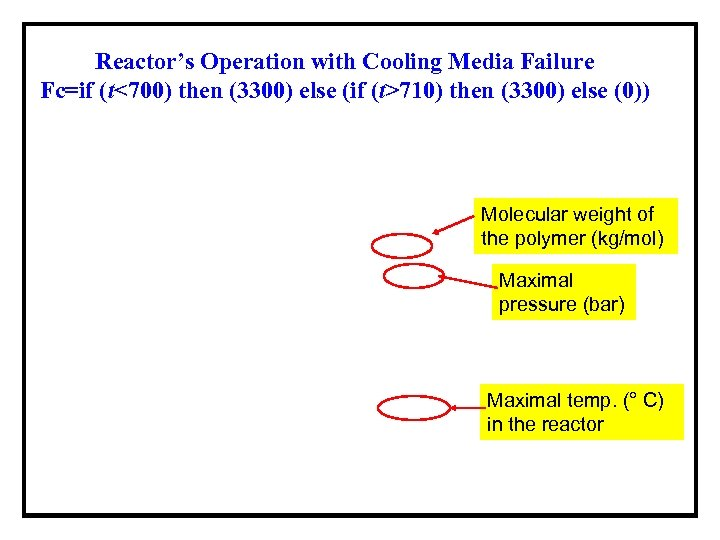Reactor’s Operation with Cooling Media Failure Fc=if (t<700) then (3300) else (if (t>710) then (3300) else (0)) Molecular weight of the polymer (kg/mol) Maximal pressure (bar) Maximal temp. (° C) in the reactor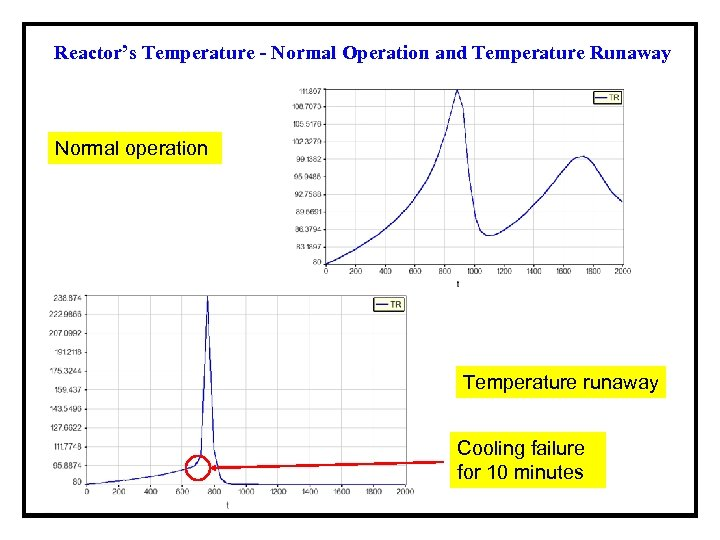Reactor’s Temperature - Normal Operation and Temperature Runaway Normal operation Temperature runaway Cooling failure for 10 minutes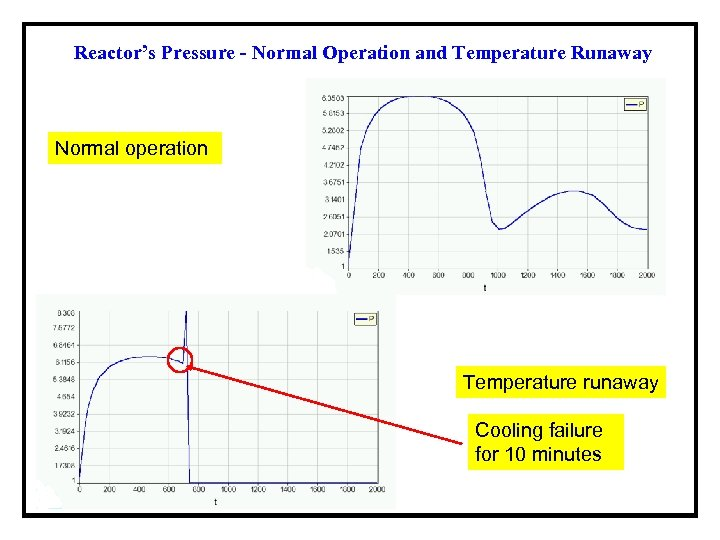Reactor’s Pressure - Normal Operation and Temperature Runaway Normal operation Temperature runaway Cooling failure for 10 minutes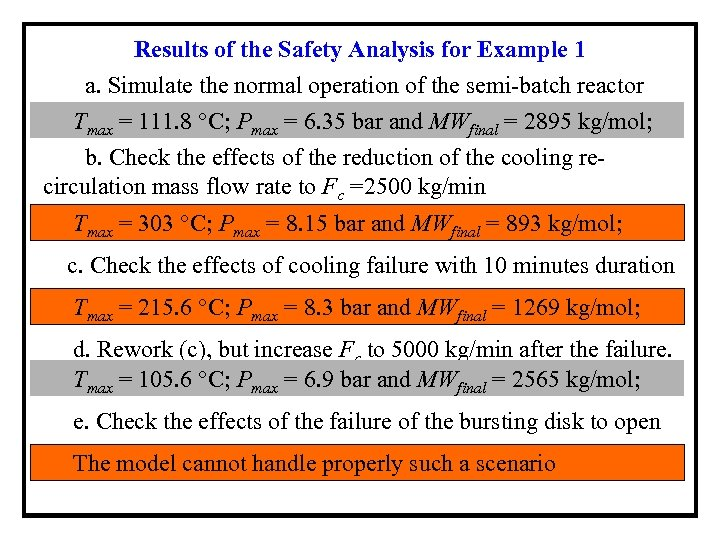Results of the Safety Analysis for Example 1 a. Simulate the normal operation of the semi-batch reactor Tmax = 111. 8 °C; Pmax = 6. 35 bar and MWfinal = 2895 kg/mol; b. Check the effects of the reduction of the cooling recirculation mass flow rate to Fc =2500 kg/min Tmax = 303 °C; Pmax = 8. 15 bar and MWfinal = 893 kg/mol; c. Check the effects of cooling failure with 10 minutes duration Tmax = 215. 6 °C; Pmax = 8. 3 bar and MWfinal = 1269 kg/mol; d. Rework (c), but increase Fc to 5000 kg/min after the failure. Tmax = 105. 6 °C; Pmax = 6. 9 bar and MWfinal = 2565 kg/mol; e. Check the effects of the failure of the bursting disk to open The model cannot handle properly such a scenario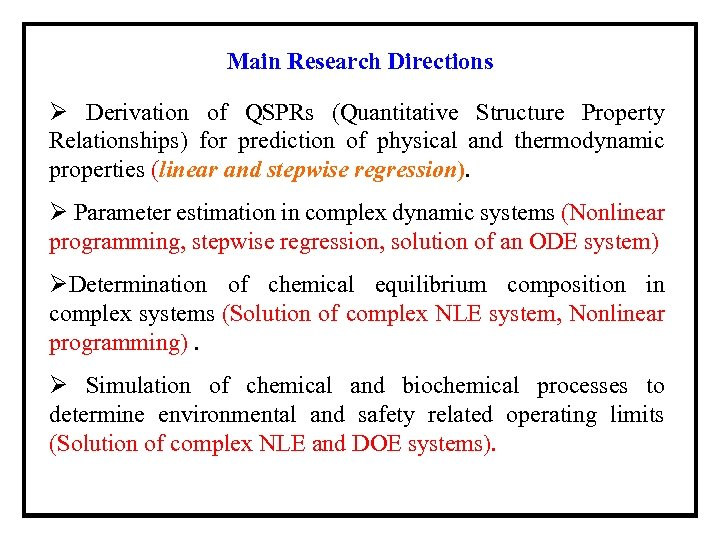Main Research Directions Ø Derivation of QSPRs (Quantitative Structure Property Relationships) for prediction of physical and thermodynamic properties (linear and stepwise regression). Ø Parameter estimation in complex dynamic systems (Nonlinear programming, stepwise regression, solution of an ODE system) ØDetermination of chemical equilibrium composition in complex systems (Solution of complex NLE system, Nonlinear programming). Ø Simulation of chemical and biochemical processes to determine environmental and safety related operating limits (Solution of complex NLE and DOE systems).